# OliNo

Renewable Energy

## LedNed spotlight MR11 WW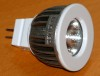presents a led lightbulb that looks like a spotlight. It is a small lightbulb and has an MR11 fitting. This article shows diverse lamp parameters, also gathered together in an Eulumdat file.

See this overview for a comparison with other light bulbs.

### Summary measurement data

parameter meas. result remark
Color temperature 2962 K Warm white
Luminous intensity Iv 151 Cd
Beam angle 13 deg
Power P 1.3 W
Power Factor 0.66 For every 1 kWh net power consumed, there has been 1.1 kVAhr for reactive power.
Luminous flux 57 lm
Luminous efficacy 44 lm/W
CRI_Ra 81 Color Rendering Index.
Coordinates chromaticity diagram x=0.4390 and y=0.4041
Fitting MR11
D x H external dimensions 35 x 43 mm External dimensions of the lightbulb.
D luminous area 20 mm Dimensions of the luminous area (used in Eulumdat file). This is equal to the size of the frontglass.
General remarks The ambient temperature during the whole set of measurements was 24-27.5 deg C.

Warm up effect: during the warming up the illuminance reduces with 8%.

Voltage dependency: the illuminance and power consumption are almost independent on the voltage (variation less than 5 %).

The Eulumdat file can be found via this link.

Measurement report (PDF)### Eulumdat light diagram

An interesting graph is the light diagram, indicating the intensity in the C0-C180 and the C90-C270 plane. This light diagram below comes from the program Qlumedit, that extracts these diagrams from an Eulumdat file.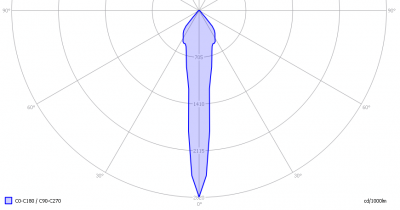The light diagram giving the radiation pattern.

It indicates the luminous intensity around the light bulb. This light bulb has symmetry over the z-axis, so the C0-C180 and C90-C270 planes show the same radiation pattern result.

The unit is Cd/1000lm, meaning the intensity in Cd assuming there would be 1000 lumen in the measured light bulb. This enables comparing different types of light bulbs.

### Illuminance Ev at 1 m distance, or luminous intensity Iv

Herewith the plot of the averaged luminous intensity Iv as a function of the inclination angle with the light bulb.The radiation pattern of the light bulb.

This radiation pattern is not the same as the one given earlier. This is because of the (small) differences of beam angles in the different planes. In this graph the luminous intensity is given in Cd.

These averaged values are used (later) to compute the lumen output.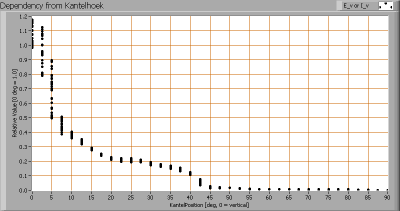Intensity data of every measured turn angle at each inclination angle.

This plot shows per inclination angle the intensity measurement results for each turn angle at that inclination angle.  There is some more spread in intensity results at low inclination angles, when the lluminance is varying a lot depending on the inclination angle.

When using the average values per inclination angle, the beam angle can be computed, being 13 degrees.

### Luminous flux

With the averaged illuminance data at 1 m distance, taken from the graph showing the averaged radiation pattern, it is possible to compute the luminous flux.

The result of this computation for this light spot is a luminous flux of 57 lm.

### Luminous efficacy

The luminous flux being 57 lm, and the power of the lightbulb being 1.3 W, yields a luminous efficacy of 44 lm/W.

A power factor of  0.66 means that for every 1 kWh net power consumed, a reactive component of 1.1 kVAr was needed.

 Light bulb voltage 11.9 V Light bulb current 164 mA Power P 1.3 W Apparent power S 2.0 VA Power factor 0.64

Of this light bulb the voltage across and the resulting current through it are measured and graphed.Voltage across and current through the lightbulb
The current has peaks; it seems that a capacitor is charged close to the tops of the voltage.
Also the power spectrum of the current is determined.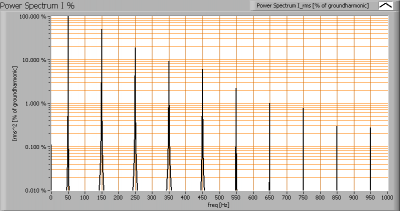The powerspectrum of the current through the light bulb.

There are higher harmonics apparent in the spectrum.

### Color temperature and Spectral power distributionThe spectral power distribution of this light bulb.

The measured color temperature is about 2950 K which is warm white.

This color temperature is measured straight underneath the light bulb. Below a graph showing the color temperature for different inclination angles.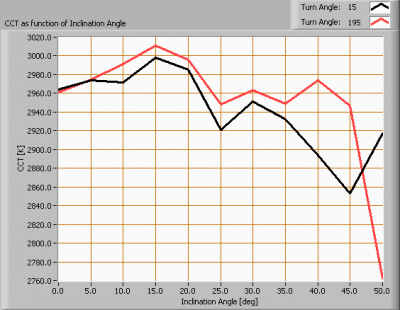Color temperature as a function of inclination angle.

The colortemperature is given for inclination angles up to 50 degrees since at larger inclination angles there is too little illumination.

The value remains constant within 4 %.

### Chromaticity diagram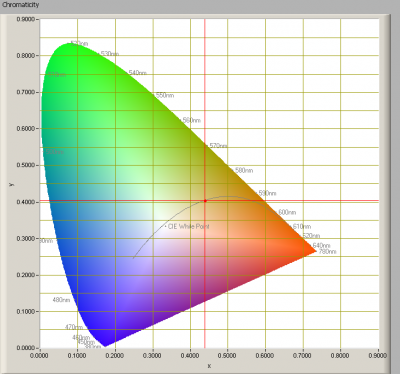The chromaticity space and the position of the lamp’s color coordinates in it.

The light coming from this lamp is on top of the Planckian Locus (the black path in the graph).

Its coordinates are x=0.4390 and y=0.4041.

### Color Rendering Index (CRI) or also Ra

Herewith the image showing the CRI as well as how well different colors are represented (rendered). The higher the number, the better the resemblance with the color when a black body radiator would have been used (the sun, or an incandescent lamp).

Each color has an index Rx, and the first 8 indexes (R1 .. R8) are averaged to compute the Ra which is equivalent to the CRI.CRI of the light of this lightbulb.

The value of 81 is higher than 80 which is considered a minimum value for indoor usage.

Note: the chromaticity difference is 0.0033 indicates the distance to the Planckian Locus. Its value is lower than 0.0054, which means that the calculated CRI result is meaningful.

### Voltage dependency

The dependency of a number of lamp parameters on the lamp voltage is determined. For this, the lamp voltage has been varied and its effect on the following lamp parameters measured: illuminance E_v [lx], color temperature CT or correlated color temperature CCT [K], the lamppower P [W] and the luminous efficacy [lm/W].Lamp voltage dependencies of certain light bulb parameters, where the value at 12 V is taken as 100 %.

The consumed power and the illuminance vary slightly when the voltage varies between 10-16 V. This variation is almost linear.

When the voltage at 12 V varies with + and – 0.25 V (comparable to +/- 5 V at 230 V), then the illuminance varies with less than + and – 0.25 %, which will not be visible when the voltage changes abruptly.

### Warm up effects

After switch on of a cold lamp, the effect of heating up of the lamp is measured on illuminance E_v [lx], color temperature CT or correlated color temperature CCT [K], the lamppower P [W] and the luminous efficacy [lm/W].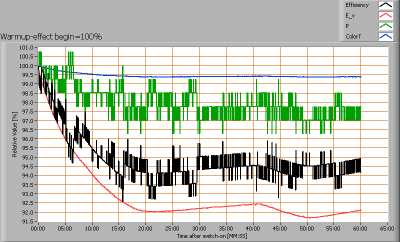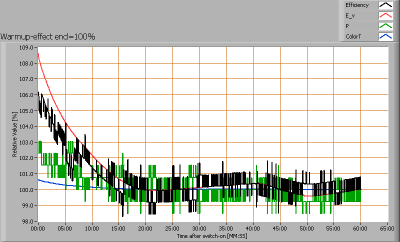Effect of warming up on different light bulb parameters. At top the 100 % level is put at begin, and at bottom at the end.

During warm up time of about 20 minutes, the light gives about 8 % less light.

The variations seen in illuminance hereafter come from the ambient temperature variations.